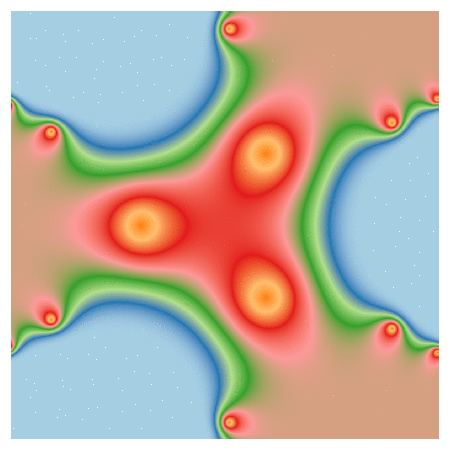# Playing With Julia (Set)

Viento, me pongo en movimiento y hago crecer las olas del mar que tienes dentro (Tercer Movimiento: Lo de Dentro, Extremoduro)

I really enjoy drawing complex numbers: it is a huge source of entertainment for me. In this experiment I play with the Julia Set, another beautiful fractal like this one. This is what I have done:

• Choosing the function `f(z)=exp(z3)-0.621`
• Generating a grid of complex numbers with both real and imaginary parts in [-2, 2]
• Iterating `f(z)` over the grid a number of times so `zn+1 = f(zn)`
• Drawing the resulting grid as I did here
• Gathering all plots into a GIF with ImageMagick as I did in my previous post: each frame corresponds to a different number of iterations

This is the result:I love how easy is doing difficult things in R. You can play with the code changing `f(z)` as well as color palettes. Be ready to get surprised:

```library(ggplot2)
library(dplyr)
library(RColorBrewer)
dir.create("output")
setwd("output")
f = function(z,c) exp(z^3)+c
# Grid of complex
z0 <- outer(seq(-2, 2, length.out = 1200),1i*seq(-2, 2, length.out = 1200),'+') %>% c()
opt <-  theme(legend.position="none",
panel.background = element_rect(fill="white"),
plot.margin=grid::unit(c(1,1,0,0), "mm"),
panel.grid=element_blank(),
axis.ticks=element_blank(),
axis.title=element_blank(),
axis.text=element_blank())
for (i in 1:35)
{
z=z0
# i iterations of f(z)
for (k in 1:i) z <- f(z, c=-0.621) df=data.frame(x=Re(z0), y=Im(z0), z=as.vector(exp(-Mod(z)))) %>% na.omit()
p=ggplot(df, aes(x=x, y=y, color=z)) +
geom_tile() +
scale_x_continuous(expand=c(0,0))+
scale_y_continuous(expand=c(0,0))+
ggsave(plot=p, file=paste0("plot", stringr::str_pad(i, 4, pad = "0"),".png"), width = 1.2, height = 1.2)
}
# Place the exact path where ImageMagick is installed
system('"C:\\Program Files\\ImageMagick-6.9.3-Q16\\convert.exe" -delay 20 -loop 0 *.png julia.gif')
# cleaning up
file.remove(list.files(pattern=".png"))
```

## 4 thoughts on “Playing With Julia (Set)”

1.Andrey Oskin says:

Concerning last two lines of your code, you may use library animation (https://cran.r-project.org/web/packages/animation/index.html), which is a nice and convenient wrapper and can save results to gif, avi etc.

“`
library(animation)
ani.options(interval = 0.2)
im.convert(‘*.png’, output = ‘julia.gif’, clean = TRUE)
“`

1.aschinchon says:

Thanks!

2.Yves Crutain says:

AAAh, my epilepsy!!
No, kidding, it is really nice 🙂
thanks!

1.aschinchon says:

Jajajajaja! Thanks!